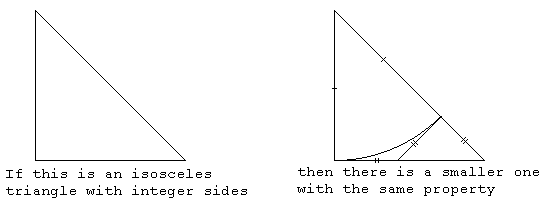Root Two Is IrrationalAllPages RecentChanges Links to this page Edit this page Search Entry portal Advice For New Users

Most, but perhaps not all, of us know that the square root of 2 can never be written exactly as a fraction. In other words,
• $\sqrt{2}$ is never a ratio of whole numbers,
• $\sqrt{2}$ is not rational,
• $\sqrt{2}$ is irrational.
How can we show this? Here are four proofs.

Algebra

The usual way is as follows.

• Suppose $\sqrt{2}$ can be written as a fraction:
• $\sqrt{2}=a/b$
• Then $2=a^2/b^2$
• Then $2b^2=a^2$
• Then $a^2$ is even
• Hence $a$ is even
• (This takes a small proof of its own.)
• So we can write a=2k
• Hence $2b^2=(2k)^2=4k^2$
• So $b^2=2k^2$
• So now $b^2$ is even
• Hence $b$ is even
• So now we can reduce $\frac{a}{b}$ to a smaller fraction.
• Lather, rinse, repeat, as they say.
• Following this process we can get smaller and smaller fractions, each of which is exactly the square root of two. Clearly this is nonsense.
• The argument is sound, so the premise must be false.
• Thus we can never write $\sqrt{2}$ as $\frac{a}{b}$

Geometry

Suppose we can draw a square that has integral sides and an integral diagonal. Using Pythagoras' theorem we can see that this is equivalent to saying that $\sqrt{2}$ is rational.

Consider the following diagram:By Pythagoras we know that if the sides are $a$ and the diagonal is $b$ then $2a^2=b^2.$ This diagram shows that given such a pair, $a,b,$ we can find a smaller pair. (Exercise for the reader: show that $a$ and $b$ in the smaller pair are still integers.)

Repeat.

Eventually we run out of numbers and thus complete our proof by contradiction

Algebra, again

Suppose $b^2=2a^2$ where $a,b$ are integers and as small as possible. Then a little algebra shows that $(2a-b)^{2}=2(b-a)^{2}$ so that $(b-a,2a-b)$ is a new pair of integers with the same property. But $b-a\lt~a$ (exercise for the reader: why?) and so we've got a smaller pair of integers whose ratio is $\sqrt{2},$ contrary to our original decision to take the smallest pair. Contradiction.

(Exercise: what's the relationship between this proof and the previous geometrical one?)

Continued Fractions

Every rational number has a finite continued fraction. We show now that the continued fraction for $1+\sqrt{2}$ does not terminate. That means $1+\sqrt{2}$ is irrational, and hence $\sqrt{2}$ is irrational.

To compute the continued fraction we separate the target number into its integer part and the fractional part

• $T=1+\sqrt{2}=2+\epsilon$ where $\epsilon=\sqrt{2}-1$
$\epsilon$ is between 0 and 1 as required, so we can take its reciprocal, giving $\frac{1}{\sqrt{2}-1}$ which after rationalising the denominator is $1+\sqrt{2}.$ That's what we started with, so the continued fraction for $1+\sqrt{2}$ is:

• $\LARGE{1+\sqrt{2}=2+\frac{1}{2+\frac{1}{2+\frac{1}{2+\frac{1}{2+...}}}}}$
Thus the continued fraction for $1+\sqrt{2}$ is [2;2,2,2,2,...] which does not terminate. Hence $1+\sqrt{2}$ is irrational, and so $\sqrt{2}$ is irrational.

CategoryMaths